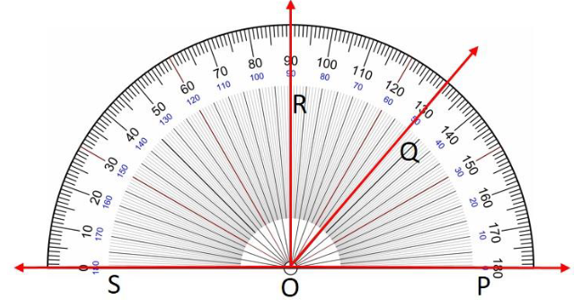Maths-
General
Easy

Question

# Use the diagram to find the measure of the indicated angle. Then classify the angle.i) ∠𝑃𝑂𝑄

## The correct answer is: 50 digres

### Step by step explanation:  Step 1: Align the protractor with the ray PO as shown above.  Start reading the inner scale from the 0°. Step 2: From the figure we can see that ray OQ is aligned on mark 50o. Hence, the measure of ∠𝑃𝑂𝑄 = 50o.∠𝑃𝑂𝑄 = 50o. Final Answer: Hence, ∠𝑃𝑂𝑄 = 50o.#### With Turito Foundation.#### Get an Expert Advice From Turito.